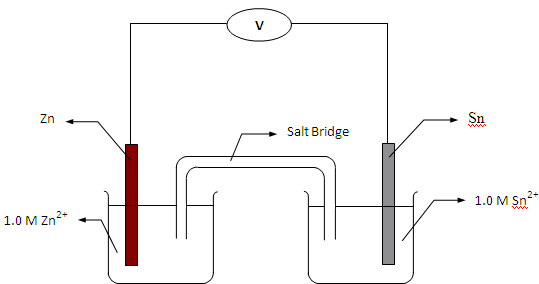## Galvanic CellsThe cell potential that is generated by a redox reactions is measured or used in a Galvanic (= electrochemical) cell. A Galvanic cell contains two compartments:
• In the oxidation part ("oxidation half cell"), a substance loses one or more electrons: "Loss of Electrons is Oxidation" (OIL)
• In the reduction part ("reduction half cell"), a substance gains one or more electrons: "Reduction Is Gain" (RIG)
The substance needing electrons in the reduction half cell pulls electrons through a wire from the substance in the oxidation half cell:
• The cell in which oxidation occurs is called the anode: oxidation at anode
• The cell in which reduction occurs is called the cathode: reduction at cathode
 The picture opposite shows a Galvanic cell made from zinc and tin half cells. The zinc half cell has a strip of zinc metal in a beaker containing Zn2+(aq) ions. The tin half cell has a strip of tin metal in a beaker containing Sn2+ ions. The two half cells are connected together with a wire and a salt bridge. The cell potential and the overall redox reaction can be worked out using the standard cell potentials. These are E° = -0.76 V for Zn2+ / Zn(s) and -0.14 V for Sn2+ / Sn(s). As the value for the Sn2+ / Sn(s) half cell is less negative, the reduction, oxidation and overall reactions are: Sn2+(aq) + 2e- → Sn(s) Zn(s) → Zn2+(aq) + 2e- Sn2+(aq) + Zn(s) → Sn(s) + Zn2+(aq)The standard cell potential is the reading on the volt meter when the cells are first connected: E°cell = E°reduction half cell - E°oxidation half cell = (-0.14 V) - (-0.76 V) = +0.62 V